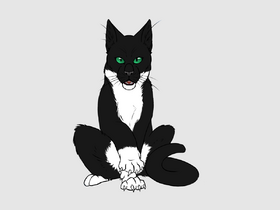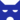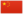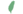—— 乌霜让雷族巡逻队带走桠爪，《雷影交加，第301页

Crowfrost影族 副族长
（继任自花楸掌 (Q2692)乌霜 Crowfrost  鴉霜

Q3211：影族公猫，自《天蚀遮月》登场的角色

## 细节

### 外貌

• 他是一只皮毛光滑、:82黑白相间的公猫。:猫物表
• Su[?]认为他有着琥珀色的眼睛。

### 勘误

• 他曾被错误地称呼为影族族长。:278

## 登场书目

• 天蚀遮月 (Q155)[猫物闲角][首次登场🐱]
• 暗夜长影 (Q156)[猫物闲角]
• 拂晓之光 (Q157)[猫物闲角]
• 第四学徒 (Q158)[猫物闲角]
• 战声渐近 (Q159)[配角]
• 暗夜密语 (Q160)[配角]
• 月光印记 (Q161)[猫物闲角]
• 武士归来 (Q162)[猫物闲角]
• 群星之战 (Q163)[对话提及]
• 学徒探索 (Q170)[配角]
• 雷影交加 (Q171)[配角][死亡时刻✝]
• 极夜无光 (Q173)[配角]
• 黑莓星的风暴 (Q182)[配角]
• 鸦羽的拷问 (Q186)[猫物闲角]
• 一星的告解 (Q4826)[配角]
• 冬青叶的故事 (Q187)[猫物闲角]
• 雾星的征兆 (Q188)[猫物闲角]
• 鸽翅的沉默 (Q192)[猫物闲角]
• 褐皮的族群 (Q3566)[仅于幻象]

• 平衡光明的黑暗 （提及）
• ## 参考文献

这篇文章基于CC BY-SA 3.0许可使用了猫武士维基（英语）Crowfrost一文中的部分内容。